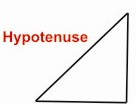HOME MATH DICTIONARY DOWNLOAD FEEDBACK DISCLAIMER
 Question: What is a Hypotenuse ? Answer: The longest side of a right angled triangle is called the hypotenuse of the triangle. The length of the hypotenuse of a right triangle can be found using the Pythagorean theorem, which states that the square of the length of the hypotenuse equals the sum of the squares of the lengths of the two other sides. For example, if one of the other sides has a length of 3 meters (when squared, 9 m²) and the other has a length of 4 m (when squared, 16 m²). Their squares add up to 25 m². The length of the hypotenuse is the square root of this, i.e. 5 m. Example of a Hypotenuse: